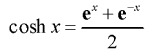# C++ cosh()

The cosh() function in C++ returns the hyperbolic cosine of an angle given in radians.

The function is defined in <cmath> header file.

`[Mathematics] cosh x = cosh(x) [In C++ Programming]`

## cosh() prototype [As of C++ 11 standard]

```double cosh(double x);
float cosh(float x);
long double cosh(long double x);
double cosh(T x); // For integral type.```

The cosh() function takes a single argument in radians and returns the hyperbolic cosine of that angle in `double`, `float` or `long double` type.

The hyperbolic cosine of x is given by,## cosh() Parameters

The cosh() function takes a single mandatory argument representing a hyperbolic angle in radians.

## cosh() Return value

The cosh() function returns the hyperbolic cosine of the argument.

If the magnitude of the result is too large to be represented by a value of the return type, the function returns `HUGE_VAL` with the proper sign, and an overflow range error occurs.

## Example 1: How cosh() function works?

``````#include <iostream>
#include <cmath>
using namespace std;
int main()
{
double x = 4.55, result;

result = cosh(x);
cout << "cosh(x) = " << result << endl;

// x in Degrees
double xDegrees = 90;
x = xDegrees * 3.14159/180;

result = cosh(x);
cout << "cosh(x) = " << result << endl;

return 0;
}``````

When you run the program, the output will be:

```cosh(x) = 47.3215
cosh(x) = 2.50918```

## Example 2: cosh() function with integral type

``````#include <iostream>
#include <cmath>
using namespace std;
int main()
{
int x = -3;
double result;

result = cosh(x);
cout << "cosh(x) = " << result << endl;

return 0;
}```
```

When you run the program, the output will be:

```cosh(x) = 10.0179
```